### Home > PC > Chapter 6 > Lesson 6.1.2 > Problem6-26

6-26.
1. When James solved cos x =he got x = cos−1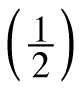. His calculator told him that
x = 1.047. Verify that this corresponds to the exact solution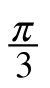. Homework Help ✎

1. We also know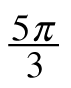is a solution to x = cos−1, but the calculator doesn’t give that answer. Why?

2. Write the complete solution to cos x =.

3. Can you get the complete solution entirely from your calculator, or do you have to draw and think?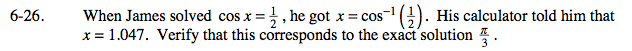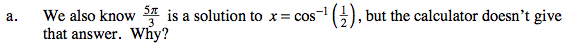There are an infinite number of solutions. The calculator chooses the solution that is in the domain of the functions inverse.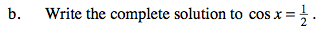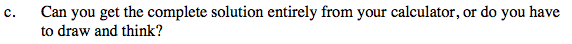Draw a unit circle and think!